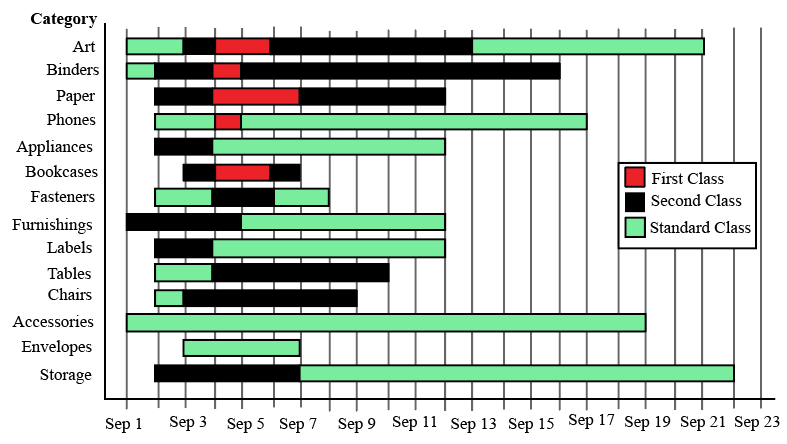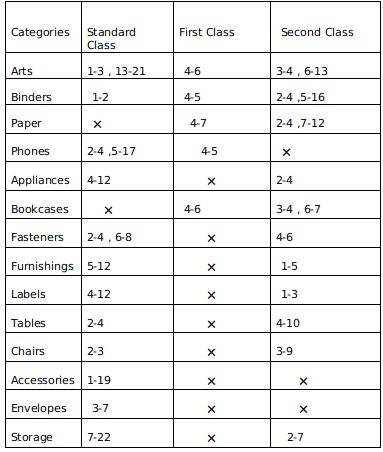### CAT 2021 Slot 2 Question 27

InstructionsThe different bars in the diagram above provide information about different orders in various categories (Art, Binders, ….) that were booked in the first two weeks of September of a store for one client. The colour and pattern of a bar denotes the ship mode (First Class / Second Class / Standard Class). The left end point of a bar indicates the booking day of the order, while the right end point indicates the dispatch day of the order. The difference between the dispatch day and the booking day (measured in terms of the number of days) is called the processing time of the order. For the same category, an order is considered for booking only after the previous order of the same category is dispatched. No two consecutive orders of the same category had identical ship mode during this period.

For example, there were only two orders in the furnishing category during this period. The first one was shipped in the Second Class. It was booked on Sep 1 and dispatched on Sep 5. The second order was shipped in the Standard class. It was booked on Sep 5 (although the order might have been placed before that) and dispatched on Sep 12. So the processing times were 4 and 7 days respectively for these orders.

Question 27

# The sequence of categories -- Art, Binders, Paper and Phones -- in decreasing order of average processing time of their orders in this period is:

Solution

Accumulating all the data :
We get the following table :Note a-b : represents the duration where a is the day when order is booked and b is the day when it is dispatched .

Now taking average processing time per order for the above mentioned categories we get :
Art =$$\frac{2+8+2+1+7}{5}\ \ =4$$
Binders =$$\frac{1+1+11+2}{4}\ \ =3.75$$
Papers = $$\frac{3+2+5}{3}\ \ =3.33$$
Phones =$$\frac{2+12+1}{3}\ \ =5$$
So in decreasing order we get Phones , Art ,Binder , Paper.